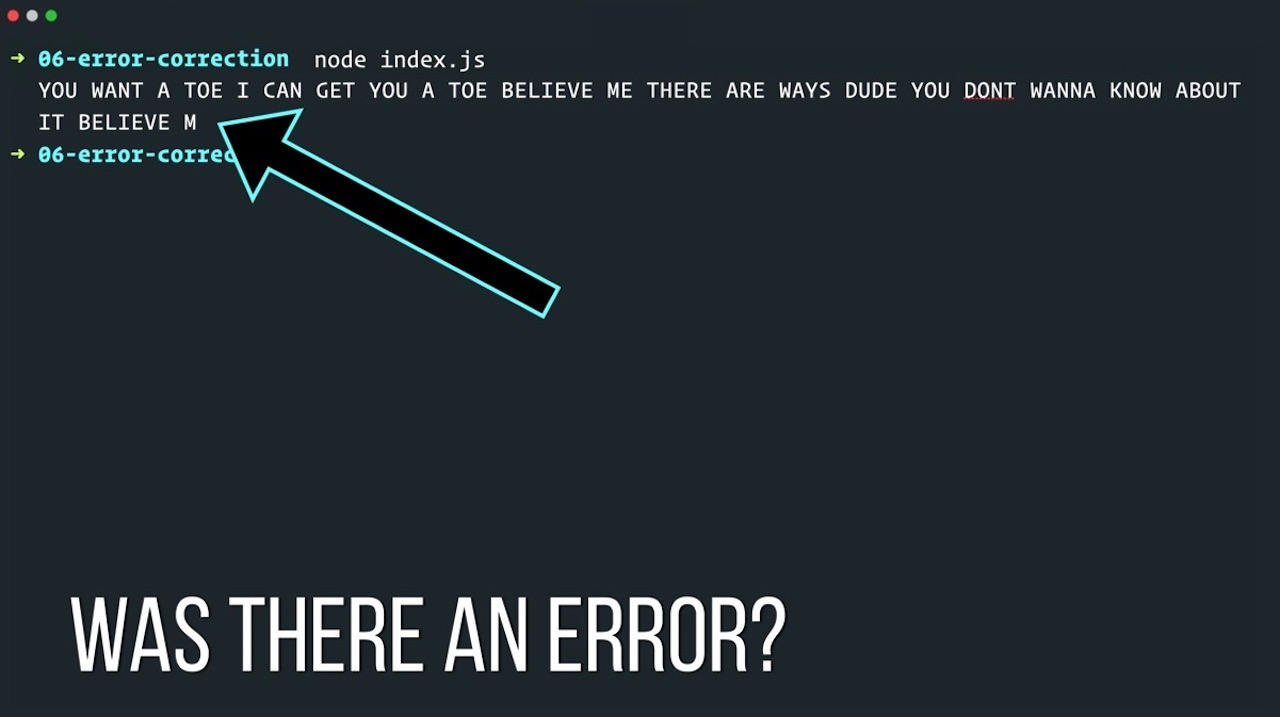👋🏼

# Error Correction, Part 1

As you watch this video, note that it's not always smooth and seamless. Behind the scenes, Vimeo (my video provider) is adjusting for bandwith changes, alternating the sources based on throughput. The player is compensating for packet loss as well, correcting for errors in audio and video.

This stuff is complicated and you're about to see why.

This is part 1 of a 2-part series where we try to answer two simple questions:

• Was there an error in the transmission?
• Where is it?

This is tricky stuff! We'll tackle the theory in this video and in the next one, part 2, we'll spend the entire time writing the code necessary to fix my favorite Big Lebowski quote.

## The Code

Here's the code used in the video. Note that the blurbs below are mostly for example. In the next video we'll write more useful stuff.

``````class Encoder{

constructor(){

this.encoding = {
"A" : "01000000000",
"B" : "10000000000",
"C" : "00100000000",
"D" : "00101000000",
"E" : "10100000000",
"F" : "10010000000",
"G" : "01010000000",
"H" : "11000000000",
"I" : "11010000000",
"J" : "01011000000",
"K" : "01011001000",
"L" : "10001000000",
"M" : "01010100000",
"N" : "01100000000",
"O" : "01110000000",
"P" : "10000100000",
"Q" : "01011000100",
"R" : "11100000000",
"S" : "11110000000",
"T" : "00110000000",
"U" : "10011000000",
"V" : "01011010000",
"W" : "00100100000",
"X" : "01011000110",
"Y" : "01011100000",
"Z" : "01011000111",
" " : "00000000000"
}
}
encode(message){
//loop the characters in the message
//we'll assume for now that they won't enter anything but alpha numeric with a space for separation
//also ... uppercase to reduce pain
const words = message.toUpperCase(), out = [];

for(let word of words){
for(let char of word){
const encoding = this.encoding[char];
//if the character can be encoded great. If not, ignore
if(encoding) out.push(encoding);
}
}
return out.join("");
}
}

evenParity(){
const keys = Object.keys(this.encoding);
for(let key of keys){
//default to even
//split into a character array so we can filter
const chars = this.encoding[key].split('');
const ones = chars.filter(v => v === "1");
//check mod 2 to see if we're odd, if so, set the pad to 1
if(ones.length % 2 !==0) pad = "1"
//update the encoding with a pad at the end
}
console.log(this.encoding);
}

checkParity(word){
//make sure we have the right length
if(word.length !== 12) return false;
//now make sure it's even
return word.split("").filter(char => char === "1").length %2 === 0;
}

decode(binaryMessage){
let out = [], codeWord = "";
for(let i = 0; i < binaryMessage.length; i+=pad){
codeWord = binaryMessage.substring(i, i + pad);
const noError = this.checkParity(codeWord);

if(noError){
const key = this.getAlpha(codeWord);
out.push(key);
codeWord = "";
}else{
//this is an error!
console.log("Got an error with ", codeWord);
const fix = this.simpleHammingErrorCorrector(codeWord);
console.log("Fixing with best guess:", fix);
out.push(fix.word);
}
}
return out.join("");
}

simpleHammingErrorCorrector(binaryMessage){

const codeWords = Object.values(this.encoding);
let bestCandidate = {}, bestDistance = Number.POSITIVE_INFINITY;

for(let codeWord of codeWords){
let thisDistance = 0;;

//we're scanning each word for a match
for(let i = 0; i < codeWord.length; i++){
if(codeWord[i] !== binaryMessage[i]) {
thisDistance+=1;
}
}
if(thisDistance < bestDistance){
bestDistance = thisDistance;
bestCandidate = {word: this.getAlpha(codeWord), binary: codeWord, distance: bestDistance}
}
}
return bestCandidate;
}
``````